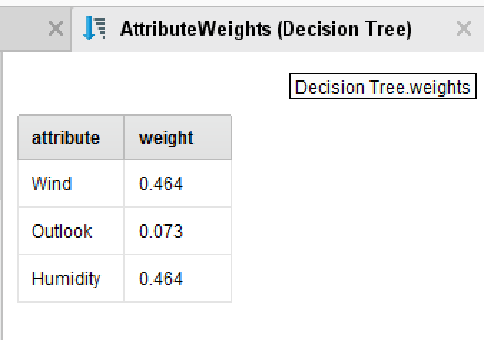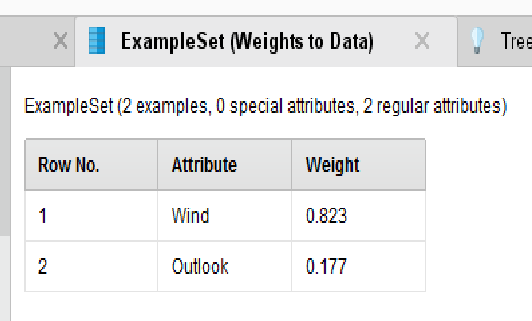# DT : different attribute weights with/without cross-validation

Moderator, RapidMiner Certified Analyst, Member Posts: 1,195Unicorn
edited November 2018 in Help

Hi,

I created 2 processes including a decision tree model from the "Golf" dataset.

1. First a classic DT model :

In this case, for the attribute weights, i get :Here the process :

`<?xml version="1.0" encoding="UTF-8"?><process version="8.0.001">  <context>    <input/>    <output/>    <macros/>  </context>  <operator activated="true" class="process" compatibility="8.0.001" expanded="true" name="Process">    <process expanded="true">      <operator activated="true" class="retrieve" compatibility="8.0.001" expanded="true" height="68" name="Retrieve Golf" width="90" x="45" y="34">        <parameter key="repository_entry" value="//Samples/data/Golf"/>      </operator>      <operator activated="true" class="concurrency:parallel_decision_tree" compatibility="8.0.001" expanded="true" height="103" name="Decision Tree" width="90" x="313" y="34"/>      <connect from_op="Retrieve Golf" from_port="output" to_op="Decision Tree" to_port="training set"/>      <connect from_op="Decision Tree" from_port="model" to_port="result 1"/>      <connect from_op="Decision Tree" from_port="exampleSet" to_port="result 2"/>      <connect from_op="Decision Tree" from_port="weights" to_port="result 3"/>      <portSpacing port="source_input 1" spacing="0"/>      <portSpacing port="sink_result 1" spacing="0"/>      <portSpacing port="sink_result 2" spacing="0"/>      <portSpacing port="sink_result 3" spacing="0"/>      <portSpacing port="sink_result 4" spacing="0"/>    </process>  </operator></process>`

2. A DT model with a cross validation :

In this case, for the attribute weights, i get :Here the process :

`<?xml version="1.0" encoding="UTF-8"?><process version="8.0.001">  <context>    <input/>    <output/>    <macros/>  </context>  <operator activated="true" class="process" compatibility="8.0.001" expanded="true" name="Process">    <process expanded="true">      <operator activated="true" class="retrieve" compatibility="8.0.001" expanded="true" height="68" name="Retrieve Golf" width="90" x="45" y="34">        <parameter key="repository_entry" value="//Samples/data/Golf"/>      </operator>      <operator activated="true" class="concurrency:cross_validation" compatibility="8.0.001" expanded="true" height="145" name="Cross Validation" width="90" x="313" y="34">        <process expanded="true">          <operator activated="true" class="concurrency:parallel_decision_tree" compatibility="8.0.001" expanded="true" height="103" name="Decision Tree" width="90" x="179" y="34"/>          <operator activated="true" class="weights_to_data" compatibility="8.0.001" expanded="true" height="68" name="Weights to Data" width="90" x="313" y="85"/>          <operator activated="true" class="remember" compatibility="8.0.001" expanded="true" height="68" name="Remember" width="90" x="447" y="85">            <parameter key="name" value="DT_weight"/>          </operator>          <connect from_port="training set" to_op="Decision Tree" to_port="training set"/>          <connect from_op="Decision Tree" from_port="model" to_port="model"/>          <connect from_op="Decision Tree" from_port="weights" to_op="Weights to Data" to_port="attribute weights"/>          <connect from_op="Weights to Data" from_port="example set" to_op="Remember" to_port="store"/>          <portSpacing port="source_training set" spacing="0"/>          <portSpacing port="sink_model" spacing="0"/>          <portSpacing port="sink_through 1" spacing="0"/>        </process>        <process expanded="true">          <operator activated="true" class="apply_model" compatibility="8.0.001" expanded="true" height="82" name="Apply Model" width="90" x="45" y="34">            <list key="application_parameters"/>          </operator>          <operator activated="true" class="performance_classification" compatibility="8.0.001" expanded="true" height="82" name="Performance" width="90" x="246" y="34">            <list key="class_weights"/>          </operator>          <connect from_port="model" to_op="Apply Model" to_port="model"/>          <connect from_port="test set" to_op="Apply Model" to_port="unlabelled data"/>          <connect from_op="Apply Model" from_port="labelled data" to_op="Performance" to_port="labelled data"/>          <connect from_op="Performance" from_port="performance" to_port="performance 1"/>          <portSpacing port="source_model" spacing="0"/>          <portSpacing port="source_test set" spacing="0"/>          <portSpacing port="source_through 1" spacing="0"/>          <portSpacing port="sink_test set results" spacing="0"/>          <portSpacing port="sink_performance 1" spacing="0"/>          <portSpacing port="sink_performance 2" spacing="0"/>        </process>      </operator>      <operator activated="true" class="recall" compatibility="8.0.001" expanded="true" height="68" name="Recall" width="90" x="447" y="136">        <parameter key="name" value="DT_weight"/>      </operator>      <connect from_op="Retrieve Golf" from_port="output" to_op="Cross Validation" to_port="example set"/>      <connect from_op="Cross Validation" from_port="model" to_port="result 3"/>      <connect from_op="Cross Validation" from_port="example set" to_port="result 2"/>      <connect from_op="Cross Validation" from_port="performance 1" to_port="result 1"/>      <connect from_op="Recall" from_port="result" to_port="result 4"/>      <portSpacing port="source_input 1" spacing="0"/>      <portSpacing port="sink_result 1" spacing="0"/>      <portSpacing port="sink_result 2" spacing="0"/>      <portSpacing port="sink_result 3" spacing="0"/>      <portSpacing port="sink_result 4" spacing="0"/>      <portSpacing port="sink_result 5" spacing="0"/>    </process>  </operator></process>`

In both cases, as expected, the two DT models are strictly the same. Why the attributes

weights are not equals ?

NB : In case of split validation, I retrieve the attribute weights of case 1.

Regards,

Lionel

Tagged:

• Administrator, Moderator, Employee, RapidMiner Certified Analyst, RapidMiner Certified Expert, Member Posts: 123RM Data Scientist
Solution Accepted

Hi Lionel,

Cross-validation will build k+1 models (k is the number of folds) and the attribute weights i.e outputted is for the last iteration. As you would know in each iteration the training set and testing sets will have different subsets of data. Hence the weight output of classic DT model (where entire data is consumed by the model at a time) and CV DT model are not same.

Also, it's always good to generate weights of the attributes using entire dataset (i.e classic model) rather than the subset of the data (i.e via cross-validation/split validation).

Cheers,

• Moderator, RapidMiner Certified Analyst, Member Posts: 1,195Unicorn

Hi Pavithra,

Thank you for this clear explanation. Now I understand better these differences of results.

So, in practice, i have to duplicate my model outside the cross-validation operator to generate

the "good weights".

Thanks you,

Best regards,

Lionel